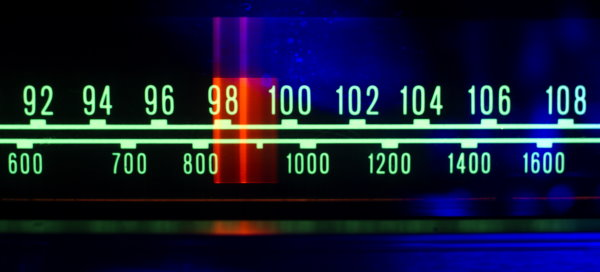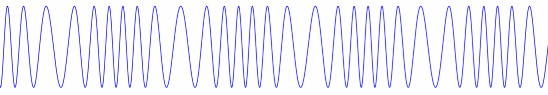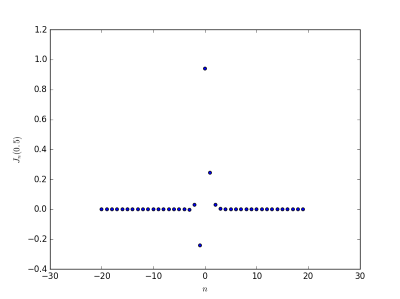# Analyzing an FM signalFrequency modulation combines a signal with a carrier wave by changing (modulating) the carrier wave’s frequency.

Starting with a cosine carrier wave with frequency fc Hz and adding a signal with amplitude β and frequency fm Hz results in the combinationThe factor β is known as the modulation index.We’d like to understand this signal in terms of cosines without any frequency modulation. It turns out the result is a set of cosines weighted by Bessel functions of β.## Component amplitudes

We will prove the equation above, but first we’ll discuss what it means for the amplitudes of the cosine components.

For small values of β, Bessel functions decay quickly, which means the first cosine component will be dominant. For larger values of β, the Bessel function values increase to a maximum then decay like one over the square root of the index. To see this we compare the coefficients for modulation index β = 0.5 and β = 5.0.

First, β = 0.5:and now for β = 5.0:For fixed β and large n we haveand so the sideband amplitudes eventually decay very quickly.

Update: See this post for what the equation above says about energy moving from the carrier to sidebands.

## Proof

To prove the equation above, we need three basic trig identitiesand three Bessel function identitiesThe Bessel function identities above can be found in Abramowitz and Stegun as equations 9.1.42, 9.1.43, and 9.1.5.and apply the sum identity for cosines to getNow let’s take the first termand apply one of our Bessel identities to expand it towhich can be simplified towhere the sum runs over all even integers, positive and negative.

Now we do the same with the second half of the cosine sum. We expandtowhich simplifies towhere again the sum is over all (odd this time) integers. Combining the two halves gives our result***

## 5 thoughts on “Analyzing an FM signal”

1. You could also take a Hilbert transform of the signal compute the instantaneous frequency (d phase(y_analytic(t))/dt) and get right back at the 2*pi*f_c + beta sin(2 pi f_m t) ;)

Which seems a little specious, but Hilbert analysis (and the Hilbert-Huang Transform, https://cds.cern.ch/record/1115835?ln=no) is great for working with a non-stationary FM signal.

2. F. Loyer

Nice article

However I would have written J2k(z) instead of Jk(z) in the first Bessel identity.

3. Javier Glz.

What would change if I want to take in acount the imaginary part of the frecuency modulation signal?

4. Janaki lokavarapu

Excellent

5. Hank Largey

Really great explanation, I think another way to examine this would be to use the Taylor series expansion of the sin in the argument, this may be a bit tedious but would better explain where the Bessel function multiplier comes from.